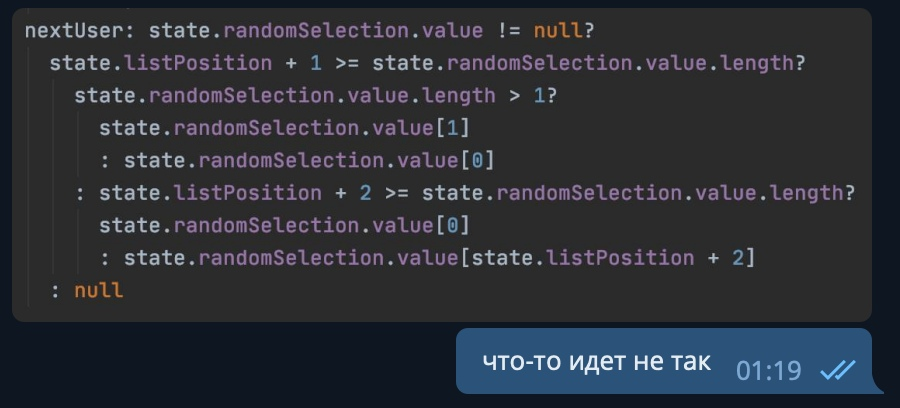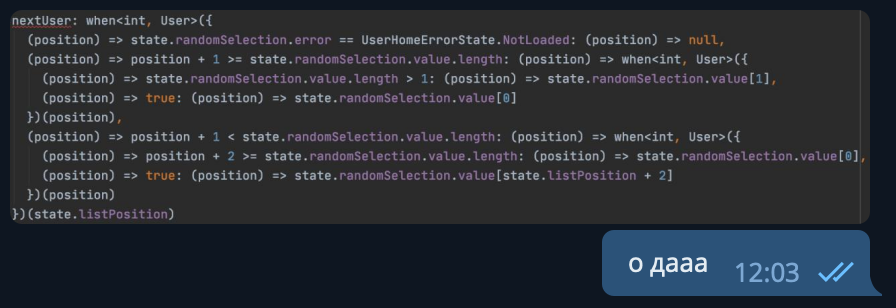# when™ `v1.0.0`

## Overview

• Wanted some functional programming in Dart?
• Realised that the only way not to use ifs and switches is ternary operatiors?

### Something has gone wrong### Now Check This!®1. Use `pub.dev` to get this s-a s-h s-n-f-w library©
``````dependencies:
when_expression: ^1.0.0
``````
1. Define `when™` statement
``````final myWhenStatement = when<int, String>({
(v) => v == 1: (_) => "a",
(v) => v == 2: (_) => "b",
(v) => v == 2: (_) => "c",
(v) => v == 4: (_) => "d",
(v) => true: (_) => "you're a failure"
});
``````
1. Be aware!
``````final myWhenStatement = when<int, String>({
(v) => v == 1: (_) => "a",
(v) => v == 2: (_) => "b", // This one will work
(v) => v == 2: (_) => "c", // This one will never be handled
(v) => v == 4: (_) => "d",
(v) => true: (_) => "you're a failure" // if your value is not handled if previous parts
// you should define else statement
});
``````
1. Call it!
``````print("out: \${myWhenStatement(1)}") // out: a
print("out: \${myWhenStatement(2)}") // out: b
print("out: \${myWhenStatement(10000)}") // out: you're a failure

//This won't compile
print("out: \${myWhenStatement('-1.0')}")
``````

## Credits:

• My righteous anger at Dart for its so-called `simplicity` (Really, you've made this wrong, congrats, dart devs!)

when_expression